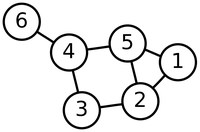# Glossary

## Graph

A graph is simply a network; as such, a graph containing a collection of nodes (or "vertices"), in which pairs of nodes are connected via segments or curves called edges. A sample graph is depicted below, in which nodes are labeled with the integers between 1 and 6.This graph is undirected, meaning that its edges do not come equipped with an orientation. For this reason, we may safely use either the notation $\{u, v\}$ or $\{v, u\}$ to denote the edge connecting nodes $u$ and $v$. This is in contrast to directed graphs, whose edges are equipped with an orientation. We may think of the edges of an undirected graph as two-way streets and those of a directed graph as one-way streets.

The abstract mathematical study of graphs and their properties is called graph theory and dates to 1735, when Leonhard Euler used a graph to demonstrate that there was no way to walk across the seven bridges of Konigsberg (in Prussia), cross each bridge exactly once, and return to one's starting point (see Eulerian cycle).